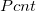# Chemical Injection Rate (Water Chemistry Control)

Use this to calculate the injection rate needed to control the chemical concentration in the irrigation water. This is common for drip irrigation to control algae growth for example. Learn more about the units used on this page. For more information go here for a fact sheet on how to calculate injection rates for water chemistry control.

Irrigation Water Flow Rate:
Desired Active Ingredient Concentration in Irrigation Water:
ppm
Product Active Ingredient Concentration (Info in chemical label):
Specific Gravity of the Chemical. (Specific gravity = chemical density / water density) (water density = 8.34 lb/gal). Info on chemical label.

Calculated Chemical Injection Rate:

## The Equation

When calculating the injection rate needed to control the chemical concentration in the irrigation water use this formula.Where= Chemical injection rate in gallons per hour (gph).= Flow rate of irrigation water in gallons per minute (gpm).= Desired chemical concentration in parts per million (ppm).= Percent of chemical in injected solution (%)= Specific Gravity of the Solution. Usually available on the label. If only chemical density is on the label can calculate as (SG = chemical density / density of water). The density of water is 1 gm/cm^3, or about 8.34 lbs/gal.

WSU Prosser - IAREC, 24106 N Bunn Rd, Prosser WA 99350-8694, 509-786-2226,Contact Us